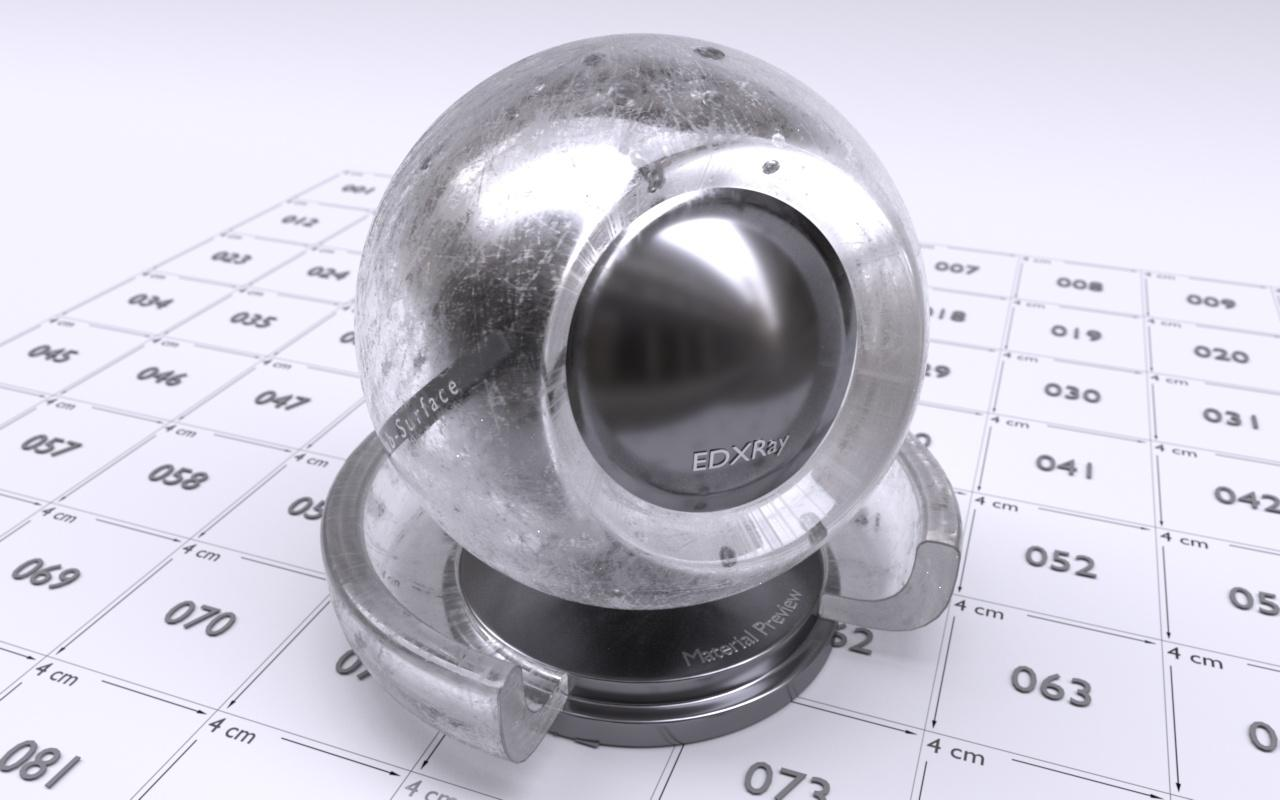Microfacet，其中// 计算镜面反射强度
float NdotH = dot(normal, Wm); // Wm = normalize(Wo + Wi)
float specularIntensity = pow(saturate( NdotH ), e);，其中代表粗糙程度。

Blinn-Phong并不是一个非常真实的分布，上面的公式也并不满足能量守恒定律（需要满足在半球面上积分为1，Blinn-Phong还需乘以一个常量才满足这个条件）。近年来有许多新的分布函数被发明出来例如Beckmann，GGX等，他们的能量分布都更接近用光学仪器测量的反射数据。Disney BRDF也使用了GGX分布。本文后面的所有渲染结果也使用了GGX。项也有各种不同的选择，本文使用了有粗糙度作为参数的Smith模型。网上GGX和Smith G项的实现很多，这里就不贴公式了，直接贴两段伪代码。

float GGX_D(Vector3 wm, float alpha) // alpha为粗糙度
{
float tanTheta2 = TanTheta2(wm),
cosTheta2 = CosTheta2(wm);

float root = alpha / (cosTheta2 * (alpha * alpha + tanTheta2));

return INV_PI * (root * root);
}

float Smith_G(Vector3 wo, Vector3 wi, Vector3 wm, float alpha)
{
auto SmithG1 = [&](Vector3 v, Vector wm)
{
float tanTheta = abs(TanTheta(v));

if (tanTheta == 0.0f)
return 1.0f;

if (Dot(v, wm) * CosTheta(v) <= 0)
return 0.0f;

float root = alpha * tanTheta;
return 2.0f / (1.0f + Sqrt(1.0f + root*root));
};

return SmithG1(wo, wm) * SmithG1(wi, wm);
}


Microfacet模型同样可以用于模拟粗糙的折射表面。顾名思义这一种材质则会将入射光线同时反射和折射到镜面反射和折射周围的方向，分布大小取决于表面的粗糙程度。

float f(Vector3 wo, Vector3 wi, Vector3 wn)
{
bool IsReflection = true;
// 根据出入射方向和法线方向判定是反射还是折射
if(Dot(wo, wn) * Dot(wi, wn) < 0.0f)
IsReflection = false;

Vector3 wm;
if(IsReflection)
wm = Normalize(wo + wi);
else // 如果是折射则根据折射率算出wm
wm = -Normalize(etai * wo + etat * wi);

float D = GGX_D(wm, Roughness);
float F = Fresnel(Dot(wo, wm), etai, etat);
float G = GGX_G(wo, wi, wm, Roughness);

// 有力D，F和G就可以套入Microfacet的公式计算当前BRDF的返回值。
}